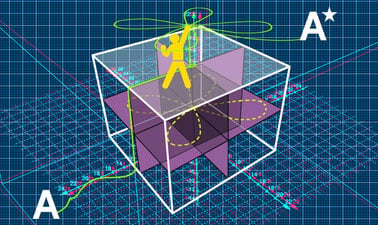A-level Further Mathematics for Year 13 - Course 1: Differential Equations, Further Integration, Curve Sketching, Complex Numbers, the Vector Product and Further Matrices

Develop your thinking skills, fluency and confidence in A-level further maths and prepare for undergraduate STEM degrees.There is one session available:

After a course session ends, it will be archivedOpens in a new tab.
Starts Jan 21
Estimated 7 weeks
2–4 hours per week
Self-paced
Free

This course by Imperial College London is designed to help you develop the skills you need to succeed in your A-level further maths exams.

You will investigate key topic areas to gain a deeper understanding of the skills and techniques that you can apply throughout your A-level study. These skills include:

* Fluency – selecting and applying correct methods to answer with speed and efficiency

* Confidence – critically assessing mathematical methods and investigating ways to apply them

* Problem solving – analysing the ‘unfamiliar’ and identifying which skills and techniques you require to answer questions

* Constructing mathematical argument – using mathematical tools such as diagrams, graphs, the logical deduction, mathematical symbols, mathematical language, construct mathematical argument and present precisely to others

* Deep reasoning – analysing and critiquing mathematical techniques, arguments, formulae and proofs to comprehend how they can be applied

Over eight modules, you will be introduced to

• Analytical and numerical methods for solving first-order differential equations
• The nth roots of unity, the nth roots of any complex number, geometrical applications of complex numbers.
• Coordinate systems and curve sketching.
• Improper integrals, integration using partial fractions and reduction formulae
• The area enclosed by a curve defined by parametric equations or polar equations, arc length and the surface area of revolution.
• Solving second-order differential equations
• The vector product and its applications
• Eigenvalues, eigenvectors, diagonalization and the Cayley-Hamilton Theorem.

Your initial skillset will be extended to give a clear understanding of how background knowledge underpins the A -level further mathematics course. You’ll also be encouraged to consider how what you know fits into the wider mathematical world.

At a glance

• Institution: ImperialX
• Subject: Math
• Level: Intermediate
• Prerequisites:

An understanding of the content of the course A-Level Further Mathematics for Year 12: Course 1 and Course 2 is required

• Language: English
• Video Transcript: English

What you'll learn

Skip What you'll learn

How to find the general or particular solution to a first-order differential equation by inspection or by using an integrating factor.

How to find a numerical solution to a differential equation using the Euler method or an improved Euler method..

How to find the nth roots of unity

How to find the nth roots of a complex number in the formHow to use complex roots of unity to solve geometrical problems.

How to identify the features of parabolas, rectangular hyperbolae, ellipses and hyperbolae defined by Cartesian and parametric equations.

How to identify features of graphs defined by rational functions.

How to define a parabola, ellipse or hyperbola using focus-directrix properties and eccentricity.

How to evaluate improper integrals.

How to integrate using partial fractions

How to derive and use reduction formulae

How to find areas enclosed by curves that are defined parametrically.

How to find the area enclosed by a polar curve.

How to calculate arc length.

How to calculate the surface area of revolution.

How to find the auxiliary equation for a second order differential equation.

Syllabus

Skip Syllabus

Module 1: First Order Differential Equations

• Solving first order differential equations by inspection
• Solving first order differential equations using an integrating factor
• Finding general and particular solutions of first-order differential equations
• Euler’s method for finding the numerical solution of a differential equation
• Improved Euler methods for solving differential equations.

Module 2: Further Complex Numbers

• The nth roots of unity and their geometrical representation
• The nth roots of a complex numberand their geometrical representation
• Solving geometrical problems using complex numbers.

Module 3: Properties of Curves

• Cartesian and parametric equations for the parabola and rectangular hyperbola, ellipse and hyperbola.
• Graphs of rational functions
• Graphs of,,for given• The focus-directrix properties of the parabola, ellipse and hyperbola, including the eccentricity.

Module 4: Further Integration Methods

• Evaluate improper integrals where either the integrand is undefined at a value in the range of integration or the range of integration extends to infinity.
• Integrate using partial fractions including those with quadratic factorsin the denominator
• Selecting the correct substitution to integrate by substitution.
• Deriving and using reduction formula

Module 5: Further Applications of Integration

• Finding areas enclosed by curves that are defined parametrically
• Finding the area enclosed by a polar curve
• Using integration methods to calculate the arc length
• Using integration methods to calculate the surface area of revolution

Module 6: Second Order Differential Equations

• Solving differential equations of form y″ + ay′ + by = 0 where a and b are constants by using the auxiliary equation.
• Solving differential equations of form y ″+ a y ′+ b y = f(x) where a and b are constants by solving the homogeneous case and adding a particular integral to the complementary function

Module 7: The Vector (cross) Product

• The definition and properties of the vector product
• Using the vector product to calculate areas of triangles.
• The vector triple product.
• Using the vector triple product to calculate the volume of a tetrahedron and the volume of a parallelepiped
• The vector product form of the vector equation of a straight line
• Solving geometrical problems using the vector product

Module 8: Matrices - Eigenvalues and Eigenvectors

• Calculating eigenvalues and eigenvectors of 2 × 2 and 3 × 3 matrices.
• Reducing matrices to diagonal form.
• Using the Cayley-Hamilton Theorem